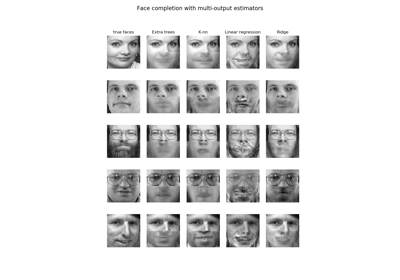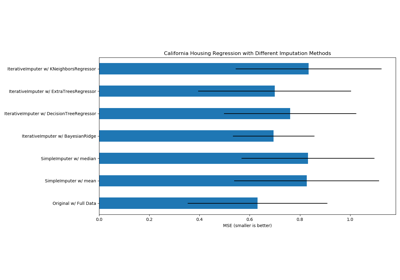# 3.2.4.3.4. sklearn.ensemble.ExtraTreesRegressor¶

class sklearn.ensemble.ExtraTreesRegressor(n_estimators=100, criterion='mse', max_depth=None, min_samples_split=2, min_samples_leaf=1, min_weight_fraction_leaf=0.0, max_features='auto', max_leaf_nodes=None, min_impurity_decrease=0.0, min_impurity_split=None, bootstrap=False, oob_score=False, n_jobs=None, random_state=None, verbose=0, warm_start=False, ccp_alpha=0.0, max_samples=None)[source]

An extra-trees regressor.

This class implements a meta estimator that fits a number of randomized decision trees (a.k.a. extra-trees) on various sub-samples of the dataset and uses averaging to improve the predictive accuracy and control over-fitting.

Read more in the User Guide.

Parameters
n_estimatorsinteger, optional (default=10)

The number of trees in the forest.

Changed in version 0.22: The default value of n_estimators changed from 10 to 100 in 0.22.

criterionstring, optional (default=”mse”)

The function to measure the quality of a split. Supported criteria are “mse” for the mean squared error, which is equal to variance reduction as feature selection criterion, and “mae” for the mean absolute error.

New in version 0.18: Mean Absolute Error (MAE) criterion.

max_depthinteger or None, optional (default=None)

The maximum depth of the tree. If None, then nodes are expanded until all leaves are pure or until all leaves contain less than min_samples_split samples.

min_samples_splitint, float, optional (default=2)

The minimum number of samples required to split an internal node:

• If int, then consider min_samples_split as the minimum number.

• If float, then min_samples_split is a fraction and ceil(min_samples_split * n_samples) are the minimum number of samples for each split.

Changed in version 0.18: Added float values for fractions.

min_samples_leafint, float, optional (default=1)

The minimum number of samples required to be at a leaf node. A split point at any depth will only be considered if it leaves at least min_samples_leaf training samples in each of the left and right branches. This may have the effect of smoothing the model, especially in regression.

• If int, then consider min_samples_leaf as the minimum number.

• If float, then min_samples_leaf is a fraction and ceil(min_samples_leaf * n_samples) are the minimum number of samples for each node.

Changed in version 0.18: Added float values for fractions.

min_weight_fraction_leaffloat, optional (default=0.)

The minimum weighted fraction of the sum total of weights (of all the input samples) required to be at a leaf node. Samples have equal weight when sample_weight is not provided.

max_featuresint, float, string or None, optional (default=”auto”)

The number of features to consider when looking for the best split:

• If int, then consider max_features features at each split.

• If float, then max_features is a fraction and int(max_features * n_features) features are considered at each split.

• If “auto”, then max_features=n_features.

• If “sqrt”, then max_features=sqrt(n_features).

• If “log2”, then max_features=log2(n_features).

• If None, then max_features=n_features.

Note: the search for a split does not stop until at least one valid partition of the node samples is found, even if it requires to effectively inspect more than max_features features.

max_leaf_nodesint or None, optional (default=None)

Grow trees with max_leaf_nodes in best-first fashion. Best nodes are defined as relative reduction in impurity. If None then unlimited number of leaf nodes.

min_impurity_decreasefloat, optional (default=0.)

A node will be split if this split induces a decrease of the impurity greater than or equal to this value.

The weighted impurity decrease equation is the following:

N_t / N * (impurity - N_t_R / N_t * right_impurity
- N_t_L / N_t * left_impurity)


where N is the total number of samples, N_t is the number of samples at the current node, N_t_L is the number of samples in the left child, and N_t_R is the number of samples in the right child.

N, N_t, N_t_R and N_t_L all refer to the weighted sum, if sample_weight is passed.

New in version 0.19.

min_impurity_splitfloat, (default=1e-7)

Threshold for early stopping in tree growth. A node will split if its impurity is above the threshold, otherwise it is a leaf.

Deprecated since version 0.19: min_impurity_split has been deprecated in favor of min_impurity_decrease in 0.19. The default value of min_impurity_split will change from 1e-7 to 0 in 0.23 and it will be removed in 0.25. Use min_impurity_decrease instead.

bootstrapboolean, optional (default=False)

Whether bootstrap samples are used when building trees. If False, the whole dataset is used to build each tree.

oob_scorebool, optional (default=False)

Whether to use out-of-bag samples to estimate the R^2 on unseen data.

n_jobsint or None, optional (default=None)

The number of jobs to run in parallel. fit, predict, decision_path and apply are all parallelized over the trees. None means 1 unless in a joblib.parallel_backend context. -1 means using all processors. See Glossary for more details.

random_stateint, RandomState instance or None, optional (default=None)

Controls 3 sources of randomness:

• the bootstrapping of the samples used when building trees (if bootstrap=True)

• the sampling of the features to consider when looking for the best split at each node (if max_features < n_features)

• the draw of the splits for each of the max_features

See Glossary for details.

verboseint, optional (default=0)

Controls the verbosity when fitting and predicting.

warm_startbool, optional (default=False)

When set to True, reuse the solution of the previous call to fit and add more estimators to the ensemble, otherwise, just fit a whole new forest. See the Glossary.

ccp_alphanon-negative float, optional (default=0.0)

Complexity parameter used for Minimal Cost-Complexity Pruning. The subtree with the largest cost complexity that is smaller than ccp_alpha will be chosen. By default, no pruning is performed. See Minimal Cost-Complexity Pruning for details.

New in version 0.22.

max_samplesint or float, default=None

If bootstrap is True, the number of samples to draw from X to train each base estimator.

• If None (default), then draw X.shape samples.

• If int, then draw max_samples samples.

• If float, then draw max_samples * X.shape samples. Thus, max_samples should be in the interval (0, 1).

New in version 0.22.

Attributes
base_estimator_ExtraTreeRegressor

The child estimator template used to create the collection of fitted sub-estimators.

estimators_list of DecisionTreeRegressor

The collection of fitted sub-estimators.

feature_importances_ndarray of shape (n_features,)

Return the feature importances (the higher, the more important the feature).

n_features_int

The number of features.

n_outputs_int

The number of outputs.

oob_score_float

Score of the training dataset obtained using an out-of-bag estimate. This attribute exists only when oob_score is True.

oob_prediction_ndarray of shape (n_samples,)

Prediction computed with out-of-bag estimate on the training set. This attribute exists only when oob_score is True.

sklearn.tree.ExtraTreeRegressor

Base estimator for this ensemble.

RandomForestRegressor

Ensemble regressor using trees with optimal splits.

Notes

The default values for the parameters controlling the size of the trees (e.g. max_depth, min_samples_leaf, etc.) lead to fully grown and unpruned trees which can potentially be very large on some data sets. To reduce memory consumption, the complexity and size of the trees should be controlled by setting those parameter values.

References

Ra7d0c8995fbc-1

P. Geurts, D. Ernst., and L. Wehenkel, “Extremely randomized trees”, Machine Learning, 63(1), 3-42, 2006.

Methods

 apply(self, X) Apply trees in the forest to X, return leaf indices. decision_path(self, X) Return the decision path in the forest. fit(self, X, y[, sample_weight]) Build a forest of trees from the training set (X, y). get_params(self[, deep]) Get parameters for this estimator. predict(self, X) Predict regression target for X. score(self, X, y[, sample_weight]) Return the coefficient of determination R^2 of the prediction. set_params(self, \*\*params) Set the parameters of this estimator.
__init__(self, n_estimators=100, criterion='mse', max_depth=None, min_samples_split=2, min_samples_leaf=1, min_weight_fraction_leaf=0.0, max_features='auto', max_leaf_nodes=None, min_impurity_decrease=0.0, min_impurity_split=None, bootstrap=False, oob_score=False, n_jobs=None, random_state=None, verbose=0, warm_start=False, ccp_alpha=0.0, max_samples=None)[source]

Initialize self. See help(type(self)) for accurate signature.

apply(self, X)[source]

Apply trees in the forest to X, return leaf indices.

Parameters
X{array-like or sparse matrix} of shape (n_samples, n_features)

The input samples. Internally, its dtype will be converted to dtype=np.float32. If a sparse matrix is provided, it will be converted into a sparse csr_matrix.

Returns
X_leavesarray_like, shape = [n_samples, n_estimators]

For each datapoint x in X and for each tree in the forest, return the index of the leaf x ends up in.

decision_path(self, X)[source]

Return the decision path in the forest.

New in version 0.18.

Parameters
X{array-like or sparse matrix} of shape (n_samples, n_features)

The input samples. Internally, its dtype will be converted to dtype=np.float32. If a sparse matrix is provided, it will be converted into a sparse csr_matrix.

Returns
indicatorsparse csr array, shape = [n_samples, n_nodes]

Return a node indicator matrix where non zero elements indicates that the samples goes through the nodes.

n_nodes_ptrarray of size (n_estimators + 1, )

The columns from indicator[n_nodes_ptr[i]:n_nodes_ptr[i+1]] gives the indicator value for the i-th estimator.

property feature_importances_
Return the feature importances (the higher, the more important the

feature).

Returns
feature_importances_array, shape = [n_features]

The values of this array sum to 1, unless all trees are single node trees consisting of only the root node, in which case it will be an array of zeros.

fit(self, X, y, sample_weight=None)[source]

Build a forest of trees from the training set (X, y).

Parameters
Xarray-like or sparse matrix of shape (n_samples, n_features)

The training input samples. Internally, its dtype will be converted to dtype=np.float32. If a sparse matrix is provided, it will be converted into a sparse csc_matrix.

yarray-like of shape (n_samples,) or (n_samples, n_outputs)

The target values (class labels in classification, real numbers in regression).

sample_weightarray-like of shape (n_samples,), default=None

Sample weights. If None, then samples are equally weighted. Splits that would create child nodes with net zero or negative weight are ignored while searching for a split in each node. In the case of classification, splits are also ignored if they would result in any single class carrying a negative weight in either child node.

Returns
selfobject
get_params(self, deep=True)[source]

Get parameters for this estimator.

Parameters
deepbool, default=True

If True, will return the parameters for this estimator and contained subobjects that are estimators.

Returns
paramsmapping of string to any

Parameter names mapped to their values.

predict(self, X)[source]

Predict regression target for X.

The predicted regression target of an input sample is computed as the mean predicted regression targets of the trees in the forest.

Parameters
Xarray-like or sparse matrix of shape (n_samples, n_features)

The input samples. Internally, its dtype will be converted to dtype=np.float32. If a sparse matrix is provided, it will be converted into a sparse csr_matrix.

Returns
yarray-like of shape (n_samples,) or (n_samples, n_outputs)

The predicted values.

score(self, X, y, sample_weight=None)[source]

Return the coefficient of determination R^2 of the prediction.

The coefficient R^2 is defined as (1 - u/v), where u is the residual sum of squares ((y_true - y_pred) ** 2).sum() and v is the total sum of squares ((y_true - y_true.mean()) ** 2).sum(). The best possible score is 1.0 and it can be negative (because the model can be arbitrarily worse). A constant model that always predicts the expected value of y, disregarding the input features, would get a R^2 score of 0.0.

Parameters
Xarray-like of shape (n_samples, n_features)

Test samples. For some estimators this may be a precomputed kernel matrix or a list of generic objects instead, shape = (n_samples, n_samples_fitted), where n_samples_fitted is the number of samples used in the fitting for the estimator.

yarray-like of shape (n_samples,) or (n_samples, n_outputs)

True values for X.

sample_weightarray-like of shape (n_samples,), default=None

Sample weights.

Returns
scorefloat

R^2 of self.predict(X) wrt. y.

Notes

The R2 score used when calling score on a regressor will use multioutput='uniform_average' from version 0.23 to keep consistent with r2_score. This will influence the score method of all the multioutput regressors (except for MultiOutputRegressor). To specify the default value manually and avoid the warning, please either call r2_score directly or make a custom scorer with make_scorer (the built-in scorer 'r2' uses multioutput='uniform_average').

set_params(self, **params)[source]

Set the parameters of this estimator.

The method works on simple estimators as well as on nested objects (such as pipelines). The latter have parameters of the form <component>__<parameter> so that it’s possible to update each component of a nested object.

Parameters
**paramsdict

Estimator parameters.

Returns
selfobject

Estimator instance.

## 3.2.4.3.4.1. Examples using sklearn.ensemble.ExtraTreesRegressor¶